2D版：

3D版：

# 什么是噪声

value_type noise(value_type p) {
...
}

# 为什么我们需要这么多噪声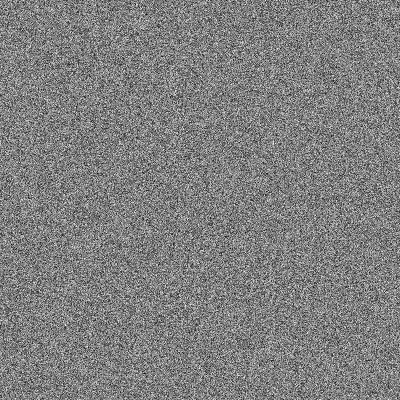# 噪声的分类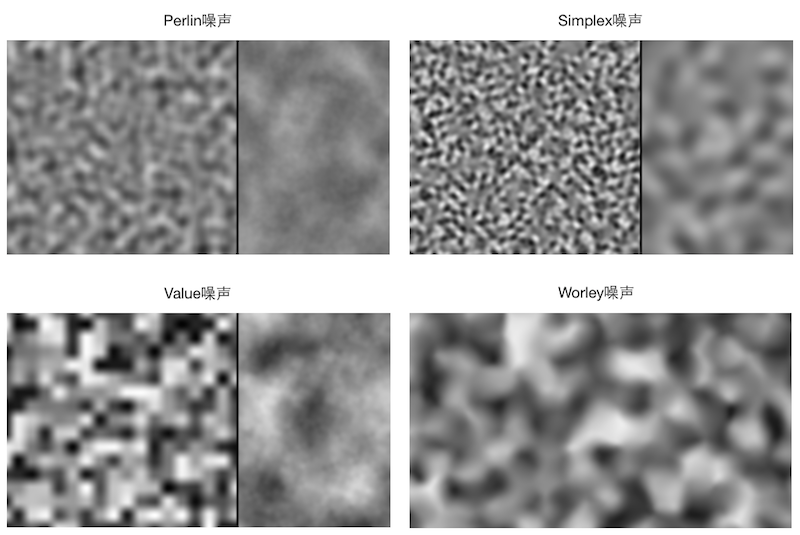Perlin噪声、Simplex噪声和Value噪声在性能上大致满足：Perlin噪声 > Value噪声 > Simplex噪声，Simplex噪声性能最好。Perlin噪声和Value噪声的复杂度是O(2n)$O(2^n)$，其中n是维数，但Perlin噪声比Value噪声需要进行更多的乘法（点乘）操作。而Simplex噪声的复杂度为O(n2)$O(n^2)$，在高纬度上优化明显。

# Perlin噪声

## 实现

Perlin噪声还是比较简单的，在1983年的计算机上实现的算法也不允许对计算量、内存有多大的要求。概括来说，Perlin噪声的实现需要三个步骤：

1. 定义一个晶格结构，每个晶格的顶点有一个“伪随机”的梯度向量（其实就是个向量啦）。对于二维的Perlin噪声来说，晶格结构就是一个平面网格，三维的就是一个立方体网格。
2. 输入一个点（二维的话就是二维坐标，三维就是三维坐标，n维的就是n个坐标），我们找到和它相邻的那些晶格顶点（二维下有4个，三维下有8个，n维下有2n$2^n$个），计算该点到各个晶格顶点的距离向量，再分别与顶点上的梯度向量做点乘，得到2n$2^n$个点乘结果。
3. 使用缓和曲线（ease curves）来计算它们的权重和。在原始的Perlin噪声实现中，缓和曲线是s(t)=3t22t3$s(t) = 3t^2-2t^3$，在2002年的论文6中，Perlin改进为s(t)=6t515t4+10t3$s(t) = 6t^5 - 15t^4 + 10t^3$。这里简单解释一下，为什么不直接使用s(t)=t$s(t) = t$，即线性插值。直接使用的线性插值的话，它的一阶导在晶格顶点处（即t = 0或t = 1）不为0，会造成明显的不连续性。s(t)=3t22t3$s(t) = 3t^2-2t^3$在一阶导满足连续性，s(t)=6t515t4+10t3$s(t) = 6t^5 - 15t^4 + 10t^3$在二阶导上仍然满足连续性。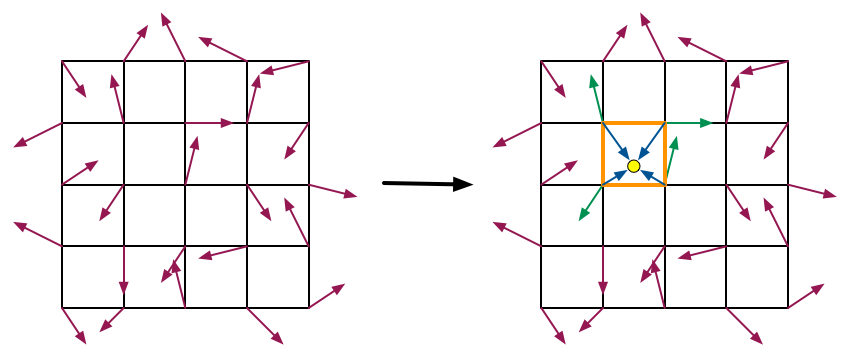G=G[(i+P[j])mod n]

## 效果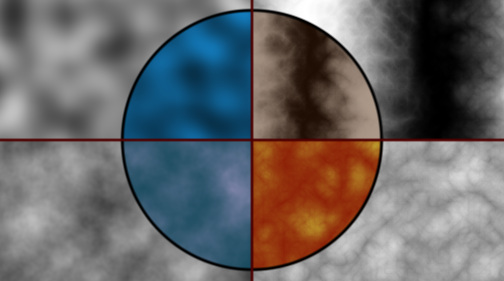vec2 hash22(vec2 p)
{
p = vec2( dot(p,vec2(127.1,311.7)),
dot(p,vec2(269.5,183.3)));

return -1.0 + 2.0 * fract(sin(p)*43758.5453123);
}
float perlin_noise(vec2 p)
{
vec2 pi = floor(p);
vec2 pf = p - pi;

vec2 w = pf * pf * (3.0 - 2.0 * pf);

return mix(mix(dot(hash22(pi + vec2(0.0, 0.0)), pf - vec2(0.0, 0.0)),
dot(hash22(pi + vec2(1.0, 0.0)), pf - vec2(1.0, 0.0)), w.x),
mix(dot(hash22(pi + vec2(0.0, 1.0)), pf - vec2(0.0, 1.0)),
dot(hash22(pi + vec2(1.0, 1.0)), pf - vec2(1.0, 1.0)), w.x),
w.y);
}

• 左上角的部分。这是最简单的单独的Perlin噪声，它的噪声模拟如下：

float noise_itself(vec2 p)
{
return noise(p * 8.0);
}

上面的代码在整个屏幕上模拟了一个8 x 8的网格结构。在绘制时，我们只需要将一个深蓝色乘以噪声即可得到类似的效果。

单独一个Perlin噪声虽然也有一定用处，但是效果往往很无趣。因此，Perlin指出可以使用不同的函数组合来得到更有意思的结果，这些函数组合通常就是指通过分形叠加（fractal sum），也就是我们之前说的fbm。

• 左下角的部分。这个部分使用了fbm进行叠加来形成一个分形噪声。公式如下：

noise(p)+12noise(2p)+14noise(4p)+...

即每一次噪声的采样频率翻倍，而振幅减少一倍。很多文章错误地把这种计算认为是Perlin噪声，这是不对的。Perlin噪声只是对应了其中每一个octave。代码是：

float noise_sum(vec2 p)
{
float f = 0.0;
p = p * 4.0;
f += 1.0000 * noise(p); p = 2.0 * p;
f += 0.5000 * noise(p); p = 2.0 * p;
f += 0.2500 * noise(p); p = 2.0 * p;
f += 0.1250 * noise(p); p = 2.0 * p;
f += 0.0625 * noise(p); p = 2.0 * p;

return f;
}

上面叠加了5层，并把初始化采样距离设置为4，这都是可以自定义的。这种噪声可以用来模拟石头、山脉这类物体。

• 右下角的部分。这一部分只是在上一部分进行了一点修改，对噪声返回值进行了取绝对值操作。它使用的公式如下：

|noise(p)|+12|noise(2p)|+14|noise(4p)|+...

它对应的代码如下：

float noise_sum_abs(vec2 p)
{
float f = 0.0;
p = p * 7.0;
f += 1.0000 * abs(noise(p)); p = 2.0 * p;
f += 0.5000 * abs(noise(p)); p = 2.0 * p;
f += 0.2500 * abs(noise(p)); p = 2.0 * p;
f += 0.1250 * abs(noise(p)); p = 2.0 * p;
f += 0.0625 * abs(noise(p)); p = 2.0 * p;

return f;
}

由于进行了绝对值操作，因此会在0值变化处出现不连续性，形成一些尖锐的效果。通过合适的颜色叠加，我们可以用这种噪声来模拟火焰、云朵这些物体。Perlin把这个公式称为turbulence（湍流？），因为它看起来挺像的。

• 右上角的部分。这个部分是在之前turbulence公式的基础上使用了一个关于表面x分量的正弦函数：

sin(x+|noise(p)|+12|noise(2p)|+14|noise(4p)|+...)

这个公式可以让表面沿着x方向形成一个条纹状的结构。Perlin使用这个公式模拟了一些大理石材质。我们的代码如下：

float noise_sum_abs_sin(vec2 p)
{
float f = 0.0;
p = p * 7.0;
f += 1.0000 * abs(noise(p)); p = 2.0 * p;
f += 0.5000 * abs(noise(p)); p = 2.0 * p;
f += 0.2500 * abs(noise(p)); p = 2.0 * p;
f += 0.1250 * abs(noise(p)); p = 2.0 * p;
f += 0.0625 * abs(noise(p)); p = 2.0 * p;
f = sin(f + p.x/32.0);

return f;
}

我们可以通过改变x分量前面的系数来控制条纹的疏密。

# Value噪声

## 实现

1. 定义一个晶格结构，每个晶格的顶点有一个“伪随机”的值（Value）。对于二维的Value噪声来说，晶格结构就是一个平面网格，三维的就是一个立方体网格。
2. 输入一个点（二维的话就是二维坐标，三维就是三维坐标，n维的就是n个坐标），我们找到和它相邻的那些晶格顶点（二维下有4个，三维下有8个，n维下有2n$2^n$个），得到这些顶点的伪随机值。
3. 使用缓和曲线（ease curves）来计算它们的权重和。同样，缓和曲线可以是s(t)=3t22t3$s(t) = 3t^2-2t^3$，也可以是s(t)=6t515t4+10t3$s(t) = 6t^5 - 15t^4 + 10t^3$（如果二阶导不连续对效果影响较大时）。

Value噪声比Perlin噪声的实现更加简单，并且需要的乘法和加法操作也更少，它只需要得到晶格顶点的随机值再把它们按权重相加即可。

## 效果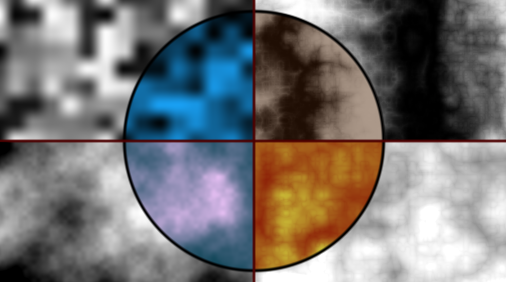float value_noise(vec2 p)
{
vec2 pi = floor(p);
vec2 pf = p - pi;

vec2 w = pf * pf * (3.0 - 2.0 * pf);

return mix(mix(hash21(pi + vec2(0.0, 0.0)), hash21(pi + vec2(1.0, 0.0)), w.x),
mix(hash21(pi + vec2(0.0, 1.0)), hash21(pi + vec2(1.0, 1.0)), w.x),
w.y);
}

# Simplex噪声

## 实现

Simplex噪声也是一种基于晶格的梯度噪声，它和Perlin噪声在实现上唯一不同的地方在于，它的晶格并不是方形（在2D下是正方形，在3D下是立方体，在更高纬度上我们称它们为超立方体，hypercube），而是单形，simplex。那么什么是单形呢？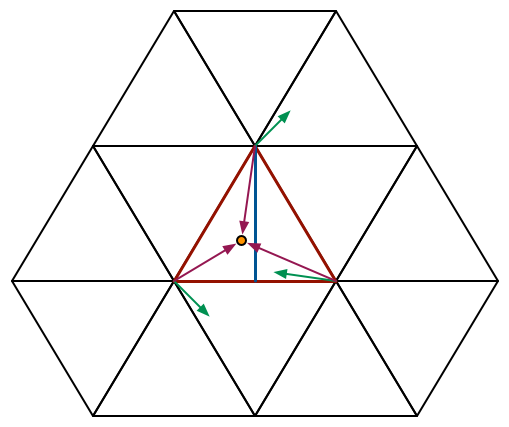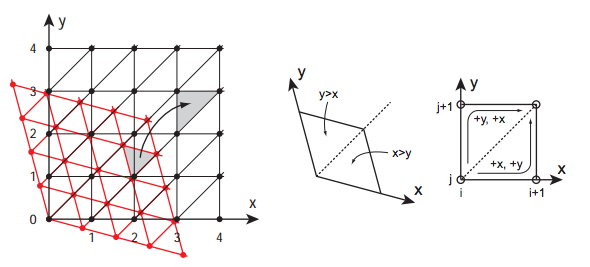x=x+(x+y+...)K1y=y+(x+y+...)K1...K1=n+11n

1 坐标偏斜：把输入点坐标进行坐标偏斜，对坐标下取整得到输入点所在的超立方体xi=floor(x),yi=floor(y),...$x_i = floor(x^{'}), y_i = floor(y^{'}), ...$，我们还可以得到小数部分xf=xxi,yf=yyi,...$x_{f} = x^{'} - x_i, y_{f} = y^{'} - y_i, ...$，这些小数部分可以帮助我们进一步判断输入点所在的单形以及计算权重。

2 单形分割：我们把之前得到的(xf,yf,...)$(x_f, y_f, ...)$中的数值按降序排序，来决定输入点位于变形后的哪个单形内。这个单形的顶点是由按序排列的(0, 0, …, 0)到(1, 1, …, 1)中的n+1$n + 1$个顶点组成，共有n!$n!$种可能性。我们可以按下面的过程来得到这n+1$n + 1$个顶点：从零坐标(0, 0, …, 0)开始，找到当前最大的分量，在该分量位置加1，直至添加了所有分量。例如，对于二维空间来说，如果xf,yf$x_f, y_f$满足xf>yf$x_f > y_f$，那么对应的3个单形坐标为：首先找到(0, 0)，由于x分量比较大，因此下一个坐标是(1, 0)，接下来是y分量，坐标为(1, 1)；对于三维空间来说，如果xf,yf,zf$x_f, y_f, z_f$满足xf>zf>yf$x_f > z_f > y_f$，那么对应的4个单形坐标位：首先从(0, 0, 0)开始，接下来在x分量上加1得(1, 0, 0)，再在z分量上加1得(1, 0, 1)，最后在y分量上加1得(1, 1, 1)。这一步的算法复杂度即为排序复杂度O(n2)$O(n^2)$

3 梯度选取：我们在偏斜后的超立方体网格上获取该单形的各个顶点的伪随机梯度向量。

4 贡献度取和：我们首先需要把单形顶点变回到之前由单形组成的单形网格。这一步需要使用第一步公式的逆函数来求得：

x=x+(x+y+...)K2y=y+(x+y+...)K2...K2=1n+11n

## 效果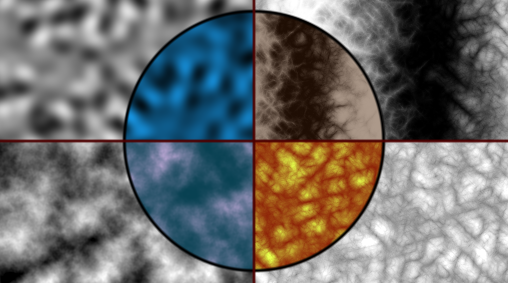float simplex_noise(vec2 p)
{
const float K1 = 0.366025404; // (sqrt(3)-1)/2;
const float K2 = 0.211324865; // (3-sqrt(3))/6;

vec2 i = floor(p + (p.x + p.y) * K1);

vec2 a = p - (i - (i.x + i.y) * K2);
vec2 o = (a.x < a.y) ? vec2(0.0, 1.0) : vec2(1.0, 0.0);
vec2 b = a - o + K2;
vec2 c = a - 1.0 + 2.0 * K2;

vec3 h = max(0.5 - vec3(dot(a, a), dot(b, b), dot(c, c)), 0.0);
vec3 n = h * h * h * h * vec3(dot(a, hash22(i)), dot(b, hash22(i + o)), dot(c, hash22(i + 1.0)));

return dot(vec3(70.0, 70.0, 70.0), n);
}

1341622170

# 可平铺的噪声

//X, Y is [0..1]
public static float SeamlessNoise( float x, float y, float dx, float dy, float xyOffset ) {
float s = x;
float t = y;

float nx = xyOffset + Mathf.Cos(s * 2.0f * Mathf.PI) * dx / (2.0f * Mathf.PI);
float ny = xyOffset + Mathf.Cos(t * 2.0f * Mathf.PI) * dy / (2.0f * Mathf.PI);
float nz = xyOffset + Mathf.Sin(s * 2.0f * Mathf.PI) * dx / (2.0f * Mathf.PI);
float nw = xyOffset + Mathf.Sin(t * 2.0f * Mathf.PI) * dy / (2.0f * Mathf.PI);

return Noise(nx, ny, nz, nw);
}

# 参考文献

1. Perlin K. Course in advanced image synthesis[C]//ACM SIGGRAPH Conference. 1984, 18.
2. Perlin K. An image synthesizer[J]. ACM Siggraph Computer Graphics, 1985, 19(3): 287-296.
3. Perlin K, Hoffert E M. Hypertexture[C]//ACM SIGGRAPH Computer Graphics. ACM, 1989, 23(3): 253-262.
4. Perlin K. Making noise[C]//Proc. of the Game Developer Conference. 1999.
5. Perlin K. Improving noise[C]//ACM Transactions on Graphics (TOG). ACM, 2002, 21(3): 681-682.
6. Perlin K. Improving noise[C]//ACM Transactions on Graphics (TOG). ACM, 2002, 21(3): 681-682.
7. Perlin K. Improving noise[C]//ACM Transactions on Graphics (TOG). ACM, 2002, 21(3): 681-682.
8. Perlin K. Making noise[C]//Proc. of the Game Developer Conference. 1999.
9. Perlin K. Noise hardware[J]. Real-Time Shading SIGGRAPH Course Notes, 2001.
10. Gustavson S. Simplex noise demystified[J]. Linköping University, Linköping, Sweden, Research Report, 2005.
11. Gustavson S. Simplex noise demystified[J]. Linköping University, Linköping, Sweden, Research Report, 2005.

05-312277

#### [图形学]仿制Minecraft游戏（柏林噪声生成地形）

06-09115

#### WebGL进阶——走进图形噪声01-06272

08-092547

#### 噪声纹理

03-295124

#### 图形学基础（4）——柏林噪声

07-3166

#### 图形学噪声解析

07-242245

#### 柏林噪声（Perlin Noise）

10-293万+

#### 【安全】Web渗透测试（全流程）

05-185715

#### 真实感计算机图形学（二）--消隐及真实感图形生成

02-132633

04-078万+

#### 超全Python图像处理讲解（多图预警）

06-131137

03-0415万+

03-11

#### HoloLens2开发入门教程

05-01©️2020 CSDN 皮肤主题: 大白 设计师: CSDN官方博客点击重新获取扫码支付1.余额是钱包充值的虚拟货币，按照1:1的比例进行支付金额的抵扣。
2.余额无法直接购买下载，可以购买VIP、C币套餐、付费专栏及课程。余额充值